Notation for Nonlinear Regression Models

This section briefly introduces the basic notation for nonlinear regression models that applies in this chapter. Additional notation is introduced throughout as needed.

The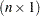vector of observed responses is denoted as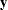. This vector is the realization of anrandom vector. The NLIN procedure assumes that the variance matrix of this random vector is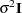. In other words, the observations have equal variance (are homoscedastic) and are uncorrelated. By defining the special variable _WEIGHT_ in your NLIN programming statements, you can introduce heterogeneous variances. If a _WEIGHT_ variable is present, then, where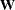is a diagonal matrix containing the values of the _WEIGHT_ variable.

The mean of the random vector is represented by a nonlinear model that depends on parametersand regressor (independent) variables: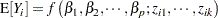In contrast to linear models, the number of regressor variables () does not necessarily equal the number of parameters () in the mean function. For example, the model fitted in the next subsection contains a single regressor and two parameters.

To represent the mean of the vector of observations, boldface notation is used in an obvious extension of the previous equation: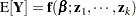The vector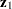, for example, is anvector of the values for the first regressor variables. The explicit dependence of the mean function onand/or thevectors is often omitted for brevity.

In summary, the stochastic structure of models fit with the NLIN procedure is mathematically captured by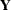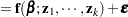Note that the residual varianceis typically also unknown. Since it is not estimated in the same fashion as the otherparameters, it is often not counted in the number of parameters of the nonlinear regression. An estimate ofis obtained after the model fit by the method of moments based on the residual sum of squares.

A matrix that plays an important role in fitting nonlinear regression models is thematrix of the first partial derivatives of the mean functionwith respect to themodel parameters. It is frequently denoted asThe use of the symbol—common in linear statistical modeling—is no accident here. The first derivative matrix plays a similar role in nonlinear regression to that of thematrix in a linear model. For example, the asymptotic variance of the nonlinear least-squares estimators is proportional to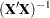, and projection-type matrices in nonlinear regressions are based on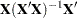. Also, fitting a nonlinear regression model can be cast as an iterative process where a nonlinear model is approximated by a series of linear models in which the derivative matrix is the regressor matrix. An important difference between linear and nonlinear models is that the derivatives in a linear model do not depend on any parameters (see previous subsection). In contrast, the derivative matrixis a function of at least one element of. It is this dependence that lies at the core of the fact that estimating the parameters in a nonlinear model cannot be accomplished in closed form, but it is an iterative process that commences with user-supplied starting values and attempts to continually improve on the parameter estimates.